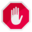## Name

ST_ShiftLongitude — Shifts the longitude coordinates of a geometry between -180..180 and 0..360.

## Synopsis

`geometry ST_ShiftLongitude(`geometry geom`)`;

## Descrizione

Reads every point/vertex in a geometry, and shifts its longitude coordinate from -180..0 to 180..360 and vice versa if between these ranges. This function is symmetrical so the result is a 0..360 representation of a -180..180 data and a -180..180 representation of a 0..360 data.This is only useful for data with coordinates in longitude/latitude; e.g. SRID 4326 (WGS 84 geographic)Pre-1.3.4 bug prevented this from working for MULTIPOINT. 1.3.4+ works with MULTIPOINT as well.Questa funzione supporta il 3d e non distrugge gli z-index.

Miglioramento nella version 2.0.0: introdotto il supporto per superfici poliedriche e TIN.

NOTE: this function was renamed from "ST_Shift_Longitude" in 2.2.0Questa funzione supporta le Polyhedral Surface.Questa funzione supporta i Triangoli e le Triangulated Irregular Network Surfaces (TIN).

## Esempi

```--single point forward transformation
SELECT ST_AsText(ST_ShiftLongitude('SRID=4326;POINT(270 0)'::geometry))

st_astext
----------
POINT(-90 0)

--single point reverse transformation
SELECT ST_AsText(ST_ShiftLongitude('SRID=4326;POINT(-90 0)'::geometry))

st_astext
----------
POINT(270 0)

--for linestrings the functions affects only to the sufficient coordinates
SELECT ST_AsText(ST_ShiftLongitude('SRID=4326;LINESTRING(174 12, 182 13)'::geometry))

st_astext
----------
LINESTRING(174 12,-178 13)
```